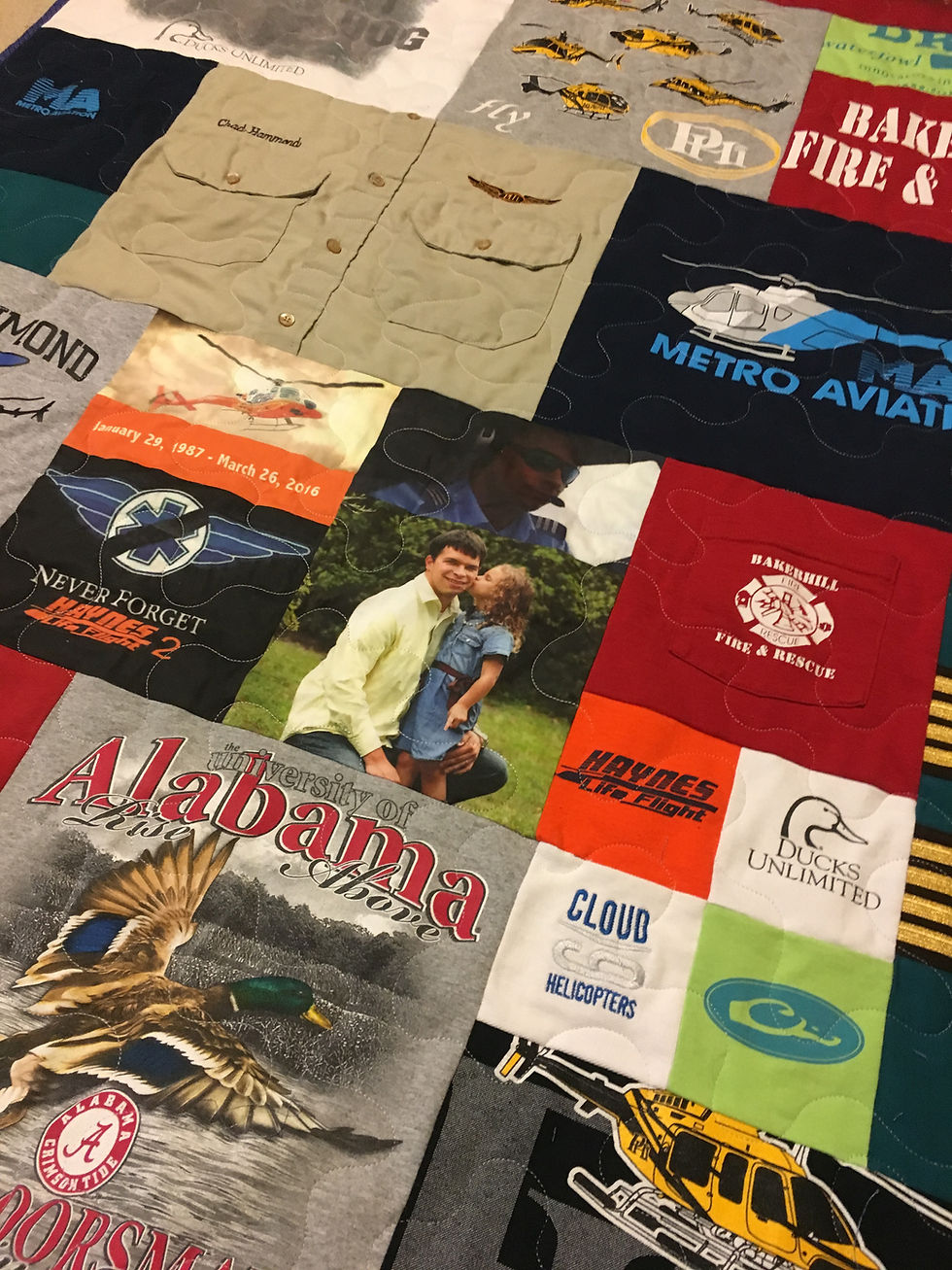top of page# Mosaic Quilt (Down Payment)

SKU: 36523641234523
\$200.00Price
`This quilt is a great way to fit LOTS of T-shirts into a quilt. With Mosaic quilts, there is more time and calculation involved. First decide on the approximate size you want to achieve then use the multipliers listed in the formulas to see an approximate cost. If you have any questions with this method, please ask.The total cost includes fabric for sashing and backing, batting, binding, and machine quilting with meandering technique. It's hard to give a price on this since it's calculated with this formula. To calculate the cost, take the length (L) plus width (W) of the quilt multiplied by the multiplierL+W=144 or less use multiplier of 3.4L+W=148 or more use multiplier of 3.656x60 quilt=60+60=120x3.4=\$40872x84 quilt=72+84=156x3.6=\$562 ADD 4" SOLID FABRIC BORDERuse multiplier of 4.0 ADD 4" and 2" SOLID FABRIC BORDERuse multiplier of 4.2`
Backing Color
bottom of page